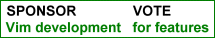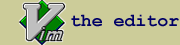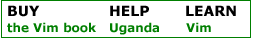bccalc.vim : evaluate equations within vim

 created by scott urban script type utility description This scirpt calculates equations using the program 'bc' (found on most linux systems, available for most systems).  Visually select the equation you want to calculate, then hit ;bc - if the selection ends with an '=' sign, the answer will be appended after the equal, otherwise, the answer is echoed as a message. Equations can span multiple lines, and the full bc syntax is probably supported.  Additionally, sin (), cos (), etc, are transformed into the names used by bc (s () c (), etc). This script was first entered as a tip, but someone pointed out that functions are hard to use when posted as tips, so this makes it easier to download and use. Here are some example lines: 2 * sqrt (2) = 3 * (2 - 1) + 4.0 ^ 6 = 4 / 3 = 3 +    4 -       2 * (1 / (3 + 2)) = define rad (x) {   return (x / 180) * 4 * atan (1) } cos (rad (45)) = ibase = 2; obase = 2; 0100 * 0101 = Select each of these in turn (continguous non-blank lines, and hit ;bc for each), and this is what you get: 2 * sqrt (2) = 2.82842712474619009760 3 * (2 - 1) + 4.0 ^ 6 = 4099.000000 4 / 3 = 1.33333333333333333333 3 +    4 -       2 * (1 / (3 + 2)) = 6.60000000000000000000 define rad (x) {   return (x / 180) * 4 * atan (1) } cos (rad (45)) = .70710678118654752440 ibase = 2; obase = 2; 0100 * 0101 = 10100 Chaining is now allowed: start with this 2 + 3 = ;bc 2 + 3 = 5 add some more stuff: 2 + 3 = 5 * 2 = ;bc 2  + 3 = 5 * 2 = 10 install details Download, place in plugin directory, or copy and paste function and mapping into your vimrc.

 rate this script Life Changing Helpful Unfulfilling

package script version date Vim version user release notes
bccalc.vim 1.9 2017-04-17 7.0 scott urban Fix map clobbering bug - now puts answer at end in multi line selection.
bccalc.vim 1.8 2005-10-28 6.0 scott urban Change to allow chaining of equations, 3 + 4 = <7> - 4 = <3> - see summary
bccalc.vim 1.7 2004-04-23 6.0 scott urban Typo
bccalc.vim 1.6 2004-04-23 6.0 scott urban Fixes for sin, cos, etc, function substitutions, thanks to C. Campbell.
Fixes for multiple substitutions.
Fix for :Calculate command
bccalc.vim 1.5 2004-04-22 6.0 scott urban Added a normal mode mapping for equation on lines by themselves - visual mode mapping can be tedious.  Added a command version, you can do
:Calculate 2 + 5 / 2 + 4
and 8 will be echoed
bccalc.vim 1.2 2002-02-18 6.0 scott urban added bccalc_preload global variable - allows specifying file(s) with functions/variables to load  before doing calculations
bccalc.vim 1.1 2002-02-16 6.0 scott urban Added "&", "<", ">", and "|" to escape characters that mess up when passed to shell.
bccalc.vim 1.0 2002-02-16 6.0 scott urban Initial upload
ip used for rating: 3.236.239.91If you have questions or remarks about this site, visit the vimonline development pages. Please use this site responsibly. Questions about Vim should go to the maillist. Help Bram help Uganda. OSDN